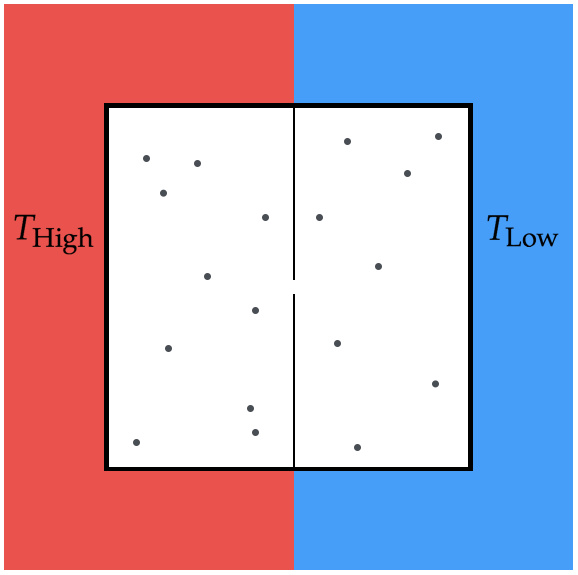# Out of the oven, into the frying panConsider a gas in the device shown above. Two halves of the box are separated by a dividing wall that has a small hole in it, which is just slightly wider than the diameter of the gas molecules. Each half of the box is in contact with a heat bath held at a given temperature.

If there are $N$ gas molecules in the box, find the expected number of gas molecules in the hot side when the box reaches steady state.

Assumptions and Details

• $N=100,000$
• $T_\text{High}=700\text{ K}$
• $T_\text{Low}=200\text{ K}$
×